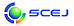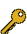\$B2=3X9)3X2qBh(B87\$BG/2q(B 2022.3.16(\$B?e(B) - 3.18(\$B6b(B) \$B?@8MBg3X(B \$BDa9CBh(B1\$B%-%c%s%Q%9(B / \$B%*%s%i%\$%s(B English page
Last Update: 2023-09-22 01:25:00

## 6-c. \$B%W%m%;%9%@%\$%J%_%/%91~MQ(B

\$B:G=*99?7F|;~!'(B2022-06-14 16:59:01\$B\$3\$NJ,N`\$G\$h\$/;H\$o\$l(B
\$B\$F\$\$\$k%-!<%o!<%I(B
\$B%-!<%o!<%I(B\$B
Packed Column Distillation1\$B7o(BComputational fluid dynamics1\$B7o(BHume flow process1\$B7o(BMass transfer1\$B7o(BTwo film theory1\$B7o(Bdynamic simulation1\$B7o(BHeat and Mass Transfer1\$B7o(BParticle flow1\$B7o(Breinforcement learning1\$B7o(BControl Volume Approach1\$B7o(BPacked distillation column1\$B7o(Bsoft sensors1\$B7o(B\$B\$BHV9f(B\$B9V1iBjL\!?H/I=\$B%-!<%o!<%I(B\$BH/I=7A<0(B
4\$B=xN1%W%m%;%9\$K4X\$9\$kM"Aw8=>]O@E*%b%G%j%s%0(B
(\$B4X@>2=3X(B) \$B!{(B(\$B@5(B)\$BJR2,(B \$BK.IW(B\$B!&(B (\$B@5(B)\$B@>B<(B \$B8aNI(B\$B!&(B (\$B@5(B)\$BLnED(B \$B=(IW(B
Packed Column Distillation
Heat and Mass Transfer
Control Volume Approach
O
382\$B@.J,7O\$N=xN1Ec\$K\$*\$1\$kJ*
(\$B4X@>2=3X(B) \$B!{(B(\$B@5(B)\$B@>B<(B \$B8aNI(B\$B!&(B (\$B@5(B)\$BJR2,(B \$BK.IW(B\$B!&(B (\$B@5(B)\$BLnED(B \$B=(IW(B\$B!&(B (\$B?@8MBg(B) (\$B@5(B)\$BBgB<(B \$BD>?M(B
Mass transfer
Packed distillation column
Two film theory
O
62\$BJ*M}%b%G%k\$H3X=,7?%7%9%F%`F1Dj\$K\$h\$k%=%U%H%;%s%5(B
(\$B;:Am8&?M9)CNG=(B/NEC) \$B!{(B(\$B@5(B)\$B7&_7(B \$B=YJ?(B\$B!&(B \$BBg@>(B \$B5.;N(B\$B!&(B (\$B;:Am8&?M9)CNG=(B/\$BElBg(B) \$BDa2,(B \$B7D2m(B
soft sensors
dynamic simulation
reinforcement learning
O
114\$B9]:`@ZCG;~\$"\$k\$\$\$OMO@\;~\$KH/@8\$9\$k(BHume\$BHyN3;R\$NHt;6N.F0%7%_%e%l!<%7%g%s(B
(\$B<(\$B@5(B)\$B2O9g(B \$B=(\$B!&(B (\$BF|@=9](B) (\$BK!(B)\$B8eF#(B \$B5A9((B\$B!&(B (\$B<\$B!&(B (\$B@5(B)\$BBg@P(B \$B5AI'(B\$B!&(B (\$BFQ>.KR9b@l(B) (\$B@5(B)\$B:4F#(B \$B?9(B\$B!&(B (\$BElKLBgB?858&(B) Stephan Siahaan Andrey Stephan\$B!&(B (\$B@5(B)\$BG8>e(B \$BMN(B
Computational fluid dynamics
Hume flow process
Particle flow
P

\$B9V1i?=\$79~\$_0lMw(B(\$B%7%s%]%8%&%`HV9f!&9V1iJ,N`HV9fJL(B)

\$B

This page was generated byeasp 2.47; update.pl 2.37 (C)1999-2015 kawase